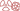THE PHENOMENON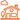IN DAILY LIFE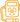QUESTION OF DOSES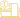AT THE DOCTOR’S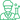At the laboratory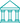At the museum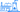NUCLEAR ENERGY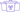A general public knowledge base dedicated to radioactivity created and maintained by the french physics community

# The electron-neutrino

## An electron that would have lost its electric charge

The easiest way to conceive a neutrino is to imagine an electron which has lost its electric charge.

For that reason, the neutrinos of radioactivity are called electron-neutrinos by physicists. This designation distinguish them from to two other kinds of neutrinos, muon-neutrinos and tau-neutrinos.

The electron-neutrino, together with the electron, is one of the two actors of beta radioactivity, an almost invisible actor, extremely difficult to detect. In the most common case of beta-minus radioactivity, it is not the neutrino which occurs instead, but its antiparticle, an electron-antineutrino. The electron-antineutrino is the partner of the positive electron or positron.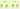Quarks and leptons
The neutrino is the fourth member of a family of four corpuscles which play a fundamental role in the world around us. The first two are the quarks “up” and “down” components of nuclear matter, the third is the electron. Physicists call leptons the pair made of the electron and the neutrino. Quarks and leptons are tiny corpuscles too small to be ever observed in a microscope. In the figure , the quarks and leptons representation by spinning tops is of course symbolic.

The electron-neutrino is the electron partner in the family of the 4 fundamental constituents of matter. The other two members of this family are the so-called up and down quarks, the elementary constituents of protons and neutrons and therefore the ultimate constituents of the atomic nucleus. Electron, electron-neutrino, quarks up and down quark form in the eyes of physicists the first generation of elementary particles.

What distinguishes the quarks from the electron and its neutrino is that the former are sensitive to the very strong interactions that cement nuclei. Thus quarks are difficult to observe because they remain captive in nuclear matter.

Electrons and neutrinos are not sensitive to the strong interaction. They take off to discover the world ! But neutrinos are furthermore electrically neutral : they do not have the electrical charge that allows electrons to form the electronic cloud that surrounds the atom nucleus. They are only sensitive to the forces responsible in particular of the beta radioactivity, that the physicists call weak interactions.

It has long been assumed that neutrinos have no mass (like photons). Recent experiments have shown that they have a extremely small mass. To give an idea, ​​the mass of the neutrino-electron is much less than the millionth of the mass of the electron, a particle already very light.

Practically massless corpuscles, neutrinos travel at the speed of light. This is how in 1987, was observed, together with a tiny flash of light coming from a distant supernova explosion, the arrival of a whiff of neutrinos … after a journey of 180,000 light years !Left and right-handed neutrinos
Neutrinos like electrons and quarks have two possible states of rotation or spin. Only one of the two states is produced : the neutrinos having a “left-handed helicity” , as indicated on the figure with respect to the direction of motion. Right-handed neutrinos are not observed: they are not produced and do not interact. In the world of antimatter is the opposite: only right antineutrinos are produced and observed. This remarkable property is a feature of the weak interaction.

Like the electrons, neutrinos have two possible states of rotation (or spin) that are called left and right-handed helicity, when the axis of rotation is chosen parrallel to the direction of motion. But neutrinos interact only through the weak forces, which distinguishes the two helicity states (a phenomenon that physicists call “parity violation“). Only left-handed neutrinos are produced and observed. Right-handed neutrinos do not interact.

Neutrinos interacting with matter only through these weak forces, their probability (called cross-section) to interact is extremely low. To give an idea of this smallness, in their experiment leading to the discovery of the neutrino in 1956, Reines and Cowan measured a cross section of 0,063 billionth of a billionth of barn.

The barn is the unity used by physicists to measure cross-sections. For instance, the cross-section of a neutron capture by a uranium-238 nucleus is of the order of a few barns. The neutrino energies in radioactive decays are generally below 1 MeV. Even the cross section of a 1 MeV neutrino , well above these Reines and Cowan measured, remains extremely low. This explains why neutrinos coming from radioactive decay occurring in the sun are able to cross through the Earth.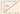An extremely low interaction probability
The neutrino probability of interaction (cross section) with a proton or neutron increases with energy. The data shown of the figure represent this interaction probability increase with energy. The interaction probability is extremely low for the neutrinos produced by radioactivity. Owing to their high energy, it is much easier to detect neutrinos foundwithin the beams of large particle accelerators.

Physicist have found two other species of neutrinos, the muon-neutrino and the tau-neutrino. The muon neutrino is the companion of the muon, a heavy electron observed in cosmic rays. The tau-neutrino is the companion of the tau, a super-heavy electron (it weighs twice as much as the proton) discovered in 1975 by the American physicist Martin Perl.

A last aspect of these fascinating particles ! Under the effect of the weak interaction, neutrinos can transform themselves into other species. Physicists say that they “oscillate”. So a neutrino born electron-neutrino in the sun can be detected as a muon-neutrino at its arrival on Earth. An observed deficit of electron-neutrino coming from the sun is one of the evidence for neutrino oscillations.THE PHENOMENONIN DAILY LIFEQUESTION OF DOSESAT THE DOCTOR’SAt the laboratoryAt the museumNUCLEAR ENERGY